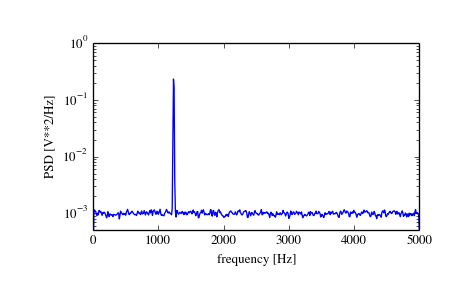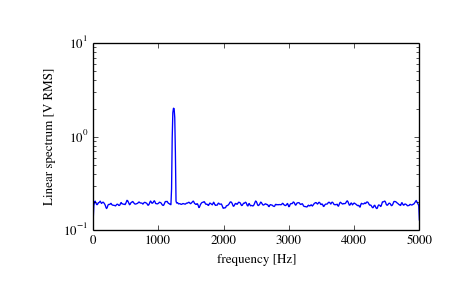# scipy.signal.welch¶

scipy.signal.welch(x, fs=1.0, window='hanning', nperseg=256, noverlap=None, nfft=None, detrend='constant', return_onesided=True, scaling='density', axis=-1)[source]

Estimate power spectral density using Welch’s method.

Welch’s method [R145] computes an estimate of the power spectral density by dividing the data into overlapping segments, computing a modified periodogram for each segment and averaging the periodograms.

Parameters: x : array_like Time series of measurement values fs : float, optional Sampling frequency of the x time series in units of Hz. Defaults to 1.0. window : str or tuple or array_like, optional Desired window to use. See get_window for a list of windows and required parameters. If window is array_like it will be used directly as the window and its length will be used for nperseg. Defaults to ‘hanning’. nperseg : int, optional Length of each segment. Defaults to 256. noverlap: int, optional Number of points to overlap between segments. If None, noverlap = nperseg / 2. Defaults to None. nfft : int, optional Length of the FFT used, if a zero padded FFT is desired. If None, the FFT length is nperseg. Defaults to None. detrend : str or function, optional Specifies how to detrend each segment. If detrend is a string, it is passed as the type argument to detrend. If it is a function, it takes a segment and returns a detrended segment. Defaults to ‘constant’. return_onesided : bool, optional If True, return a one-sided spectrum for real data. If False return a two-sided spectrum. Note that for complex data, a two-sided spectrum is always returned. scaling : { ‘density’, ‘spectrum’ }, optional Selects between computing the power spectral density (‘density’) where Pxx has units of V**2/Hz if x is measured in V and computing the power spectrum (‘spectrum’) where Pxx has units of V**2 if x is measured in V. Defaults to ‘density’. axis : int, optional Axis along which the periodogram is computed; the default is over the last axis (i.e. axis=-1). f : ndarray Array of sample frequencies. Pxx : ndarray Power spectral density or power spectrum of x.

periodogram
Simple, optionally modified periodogram
lombscargle
Lomb-Scargle periodogram for unevenly sampled data

Notes

An appropriate amount of overlap will depend on the choice of window and on your requirements. For the default ‘hanning’ window an overlap of 50% is a reasonable trade off between accurately estimating the signal power, while not over counting any of the data. Narrower windows may require a larger overlap.

If noverlap is 0, this method is equivalent to Bartlett’s method [R146].

New in version 0.12.0.

References

 [R145] (1, 2) P. Welch, “The use of the fast Fourier transform for the estimation of power spectra: A method based on time averaging over short, modified periodograms”, IEEE Trans. Audio Electroacoust. vol. 15, pp. 70-73, 1967.
 [R146] (1, 2) M.S. Bartlett, “Periodogram Analysis and Continuous Spectra”, Biometrika, vol. 37, pp. 1-16, 1950.

Examples

```>>> from scipy import signal
>>> import matplotlib.pyplot as plt
```

Generate a test signal, a 2 Vrms sine wave at 1234 Hz, corrupted by 0.001 V**2/Hz of white noise sampled at 10 kHz.

```>>> fs = 10e3
>>> N = 1e5
>>> amp = 2*np.sqrt(2)
>>> freq = 1234.0
>>> noise_power = 0.001 * fs / 2
>>> time = np.arange(N) / fs
>>> x = amp*np.sin(2*np.pi*freq*time)
>>> x += np.random.normal(scale=np.sqrt(noise_power), size=time.shape)
```

Compute and plot the power spectral density.

```>>> f, Pxx_den = signal.welch(x, fs, nperseg=1024)
>>> plt.semilogy(f, Pxx_den)
>>> plt.ylim([0.5e-3, 1])
>>> plt.xlabel('frequency [Hz]')
>>> plt.ylabel('PSD [V**2/Hz]')
>>> plt.show()
```If we average the last half of the spectral density, to exclude the peak, we can recover the noise power on the signal.

```>>> np.mean(Pxx_den[256:])
0.0009924865443739191
```

Now compute and plot the power spectrum.

```>>> f, Pxx_spec = signal.welch(x, fs, 'flattop', 1024, scaling='spectrum')
>>> plt.figure()
>>> plt.semilogy(f, np.sqrt(Pxx_spec))
>>> plt.xlabel('frequency [Hz]')
>>> plt.ylabel('Linear spectrum [V RMS]')
>>> plt.show()
```The peak height in the power spectrum is an estimate of the RMS amplitude.

```>>> np.sqrt(Pxx_spec.max())
2.0077340678640727
```

#### Previous topic

scipy.signal.periodogram

#### Next topic

scipy.signal.lombscargle